Courses

Test: MCQs (One or More Correct Option): Waves | JEE Advanced

25 Questions MCQ Test Physics 35 Years JEE Main & Advanced Past year Papers | Test: MCQs (One or More Correct Option): Waves | JEE Advanced

Description
Attempt Test: MCQs (One or More Correct Option): Waves | JEE Advanced | 25 questions in 50 minutes | Mock test for JEE preparation | Free important questions MCQ to study Physics 35 Years JEE Main & Advanced Past year Papers for JEE Exam | Download free PDF with solutions
*Multiple options can be correct
QUESTION: 1

A wave equation which gives the displacement along the y-direction is given by y = 10–4 sin (60t + 2x) where x and y are in metres and t is time in seconds. This represents a wave

Solution:

y = 10–4 sin (60t + 2x)
Comparing the given equation with the standard wave equation travelling in negative x-direction
y = a sin (ωt + k x) we get amplitude a = 10–4m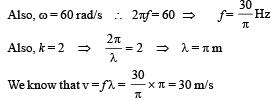*Multiple options can be correct
QUESTION: 2

A transver se wave is described by the equation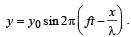The maximum particle velocity is equal to four times the wave velocity if

Solution: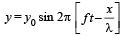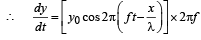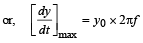Given that the maximum particle velocity is equal to four times the wave velocity (c = f × λ)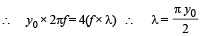*Multiple options can be correct
QUESTION: 3

An air column in a pipe, which is closed at one end, will be in resonance with a vibrating tuning fork of frequency 264 Hz if the length of the column in cm is :

Solution:

The wavelengths possible in an air column in a pipe which has one closed end is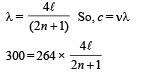n = 264 Hz as it is in resonance with a vibrating turning fork of frequency 264 Hz.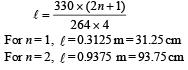*Multiple options can be correct
QUESTION: 4

A tube, closed at one end and containing air, produces, when excited, the fundamental note of frequency 512 Hz. If the tube is open at both ends the fundamental frequency that can be excited is (in Hz)

Solution: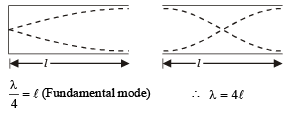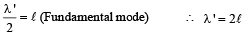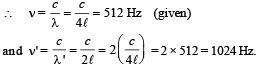*Multiple options can be correct
QUESTION: 5

The displacement of particles in a string stretched in the x-direction is represented by y. Among the following expressions for y, those describing wave motion are :

Solution:

For wave motion , the differential equation is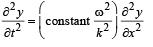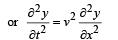....(i)

NOTE : The wave motion is characterized by the two conditions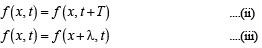*Multiple options can be correct
QUESTION: 6

An organ pipe P1 closed at one end vibrating in its first harmonic and another pipe P2 open at ends vibrating in its third harmonic are in resonance with a given tuning fork.
The ratio of the length of P1 to that of P2 is

Solution: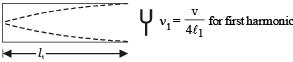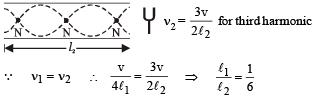*Multiple options can be correct
QUESTION: 7

Velocity of sound in air is 320 m/s. A pipe closed at one end has a length of 1 m. Neglecting end corrections, the air column in the pipe can resonate for sound of frequency :

Solution: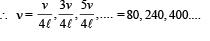*Multiple options can be correct
QUESTION: 8

A wave is represented by the equation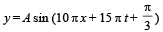where x is in meters and t is in seconds. The expression represents :

Solution:

y = A sin (10 πx + 15 πt + π/3)

The standard equation of a wave travelling in – X direction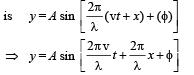Comparing it with the given equation, we find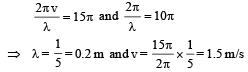*Multiple options can be correct
QUESTION: 9

Two identical straight wires are stretched so as to produce 6 beats per second when vibrating simultaneously. On changing the tension slightly in one of them, the beat frequency remains unchanged. Denoting by T1 , T2 the higher and the lower initial tension in the strings, then it could be said that while making the above changes in tension,

Solution: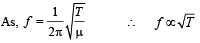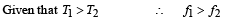Initially beat frequency (f1 - f2) = 6.
The beat frequency remains unchanged which is possible when f2 increases and f1 decreases. Thus T2 increases and T1  decreases.

*Multiple options can be correct
QUESTION: 10

The displacement y of a particle executing periodic motion is given by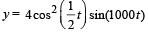This expression may be considereed to be a result of the superposition of

Solution: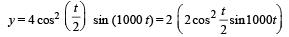= 2 [cos t + 1] sin 1000t
= 2 cos t sin 1000t + 2 sin 1000t
= sin 1000t + sin 999t + 2 sin 1000t

*Multiple options can be correct
QUESTION: 11

A sound wave of frequency f travels horizontally to the right. It is reflected from a large vertical plane surface moving to left with a speed v. The speed of sound in medium is C

Solution:

Frequency of reflected wave is f ''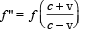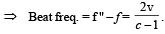Wavelength of reflected wave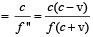*Multiple options can be correct
QUESTION: 12

A string of length 0.4 m and mass 10–2 kg is tightly clamped at its ends. The tension in the string is 1.6 N. Identical wave pulses are produced at one end at equal intervals of time, Δt. The minimum value of Δt which allows constructive interference between successive pulses is

Solution:

KEY CONCEPT : The time required for constructive interference  equal to the time period of a wave pulse.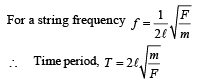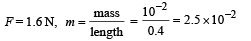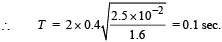*Multiple options can be correct
QUESTION: 13

The (x, y) co-ordinates of the corners of a square plate are (0, 0), (L, 0), (L, L) and (0, L). The edges of the plate are clamped and transverse standing waves are set up in it. If u(x, y) denotes the displacement of the plate at the point (x, y) at some instant of time, the possible expression(s) for u is (are)  (a = positive constant)

Solution:

Due to the clamping of the square plate at the edges, its displacements along the x and y axes will individually be zero at the edges. Only the choices (b) and (c) predict these displacements correctly. This is because sin 0 = 0.
Option (a) :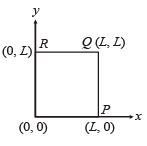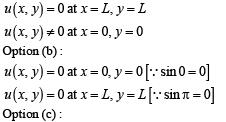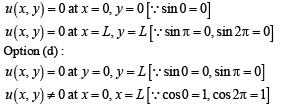*Multiple options can be correct
QUESTION: 14

A transverse sinusoidal wave of amplitude a, wavelength l and frequency f is travelling on a stretched string. The maximum speed of any point on the string is v/10, where v is the speed of propagation of the wave. If a = 10–3m and v = 10 m s–1, then λ and f are given by

Solution:

NOTE : For a transverse sinusodial wave travelling on a string, the maximum velocity is aω.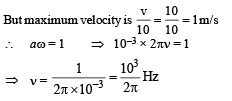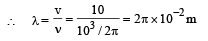*Multiple options can be correct
QUESTION: 15

y(x, t) = 0.8/[4x+5t)2+5] represents a moving pulse, where x and y are in meter and t in second. Then

Solution: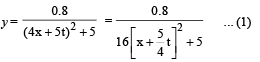We know that equation of moving pulse is

y = f (x + vt)       ... (2)

On comparing (1)  and (2), we get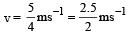So, the wave will travel a distance of 2.5 m in 2 sec.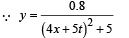At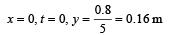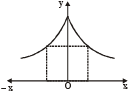∴ maximum displacement is 0.16 m

The graph for the given equation is drawn. This is symmetric about y-axis.

*Multiple options can be correct
QUESTION: 16

In a wave motion y = a sin (kx - ωt), y can represent

Solution:

In the wave motion y = a (kx - ωt) , y can represent electric and magnetic fields in electromagnetic waves and displacement and pressure in sound waves.

*Multiple options can be correct
QUESTION: 17

Standing waves can be produced

Solution:

Standing waves are produced by two similar waves superposing while travelling in opposite direction.
This can happen in case (a), (b) and (c).

*Multiple options can be correct
QUESTION: 18

As a wave propagates,

Solution:

For a plane wave, intensity (energy crossing per unit area  per unit time) is constant at all points.
But for a spherical wave, intensity at a distance r from a point source of power (P), is given by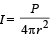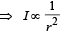But the total intensity of the spherical wave over the spherical surface centered at the source remains constant at all times.

NOTE :  For line source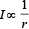*Multiple options can be correct
QUESTION: 19

A student performed the experiment to measure the speed of sound in air using resonance air-column method. Two resonances in the air-column were obtained by lowering the water level. The resonance with the shorter air-column is the first resonance and that with the longer air-column is the second resonance. Then,

Solution:

At second resonance the length of air column is more as compared to first resonance. Now, longer the length of air column, more is the absorption of energy and lesser is the intensity of sound heard.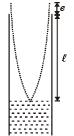As shown in the figure, the length of the air column at the first resonance is somewhat shorter than 1/4 th of the wavelength of the sound in air due to end correction.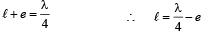*Multiple options can be correct
QUESTION: 20

A person blows into open-end of a long pipe. As a result, a high pressure pulse of air travels down the pipe.
When this pulse reaches the other end of the pipe,

Solution:

When sound pulse is reflected through a rigid boundary (closed end of a pipe), no phase change occurs between the incident and reflected pulse i.e., a high pressure pulse is reflected as a high pressure pulse.
When a sound pulse is reflected from open end of a pipe, a phase change of a radian occurs between the incident and the reflected pulse. A high pressure pulse is reflected as a low pressure pulse.

*Multiple options can be correct
QUESTION: 21

A horizontal stretched string, fixed at two ends, is vibrating in its fifth harmonic according to the equation, y(x, t) = (0.01 m) sin [(62.8 m–1)x] cos[(628 s–1)t]. Assuming π = 3.14, the correct statement(s) is (are)

Solution:

y = [0.01 sin (62.8x)] cos (628 t).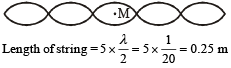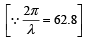The midpoint M is an antinode and has the maximum displacement = 0.01 m

The fundamental frequency =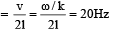*Multiple options can be correct
QUESTION: 22

Two vehicles, each moving with speed u on the same horizontal straight road, are approaching each other. Wind blows along the road with velocity w. One of these vehicles blows a whistle of frequency f1. An observer in the other vehicle hears the frequency of the whistle to be f2. The speed of sound in still air is V. The correct statement(s) is (are)

Solution: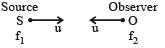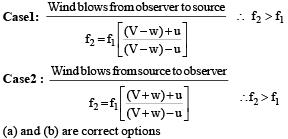*Multiple options can be correct
QUESTION: 23

A student is performing an experiment using a resonance column and a tuning fork of frequency 244 s–1. He is told that the air in the tube has been replaced by another gas (assume that the column remains filled with the gas). If the minimum height at which resonance occurs is (0.350 ± 0.005) m, the gas in the tube is

(Useful information: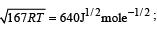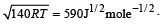The molar masses M in grams are given in the options. Take the values of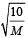for eachgas as given there.)

Solution: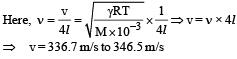For monatomic gas γ =1.67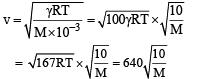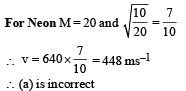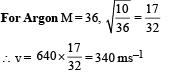∴ (d) is the correct option.
For diatomic gas γ = 1.4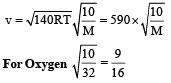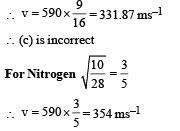∴ (b)  is incorrect

*Multiple options can be correct
QUESTION: 24

One end of a taut string of length 3 m along the x-axis is fixed at x = 0. The speed of the waves in the string is 100 ms–1. The other end of the string is vibrating in the y-direction so that stationary waves are set up in the string. The possible waveform (s) of these stationary waves is(are)

Solution:

Clearly in the given situation a displacement node is present at x = 0 and a displacement antinode is present at x = 3 m.
Therefore, y = 0 at x = 0 and y =±A at x = 3 m.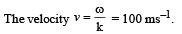a, c and d are the correct options which satisfy the above conditions.

*Multiple options can be correct
QUESTION: 25

Two loudspeakers M and N are located 20 m apart and emit sound at frequencies 118 Hz and 121 Hz, respectively. A car is initially at a point P , 1800 m away from the midpoint Q of the line MN and moves towards Q constantly at 60 km/hr along the perpendicular bisector of MN. It crosses Q and eventually reaches a point R, 1800 m away from Q. Let v(t) represent the beat frequency measured by a person sitting in the car at time t. Let vP , vQ and vR be the beat frequencies measured at locations P, Q and R, respectively. The speed of sound in air is 330 ms–1. Which of the following statement(s) is(are) true regarding the sound heard by the person?

Solution: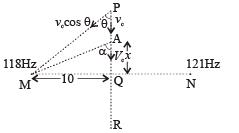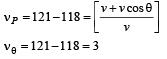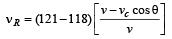∴ nP + nR = 2 nQ ⇒ (A) is correct option
In general when the car is passing through A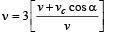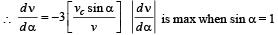i.e.,   α = 90° (at Q)
⇒ (b) is correct option.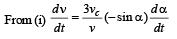… (ii)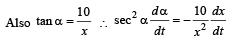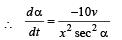…(iii)
From (ii) & (iii)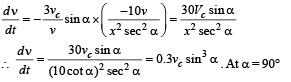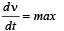∴ (c) is the correct optionUse Code STAYHOME200 and get INR 200 additional OFF Use Coupon Code

Track your progress, build streaks, highlight & save important lessons and more!

Similar ContentRelated tests